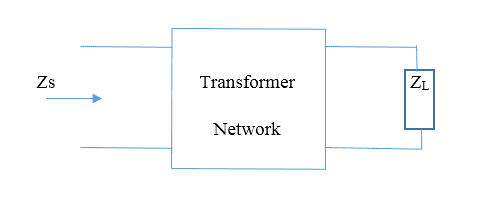### Quick Contact# How to Enable Impedance Transformation

Impedance transformation is a crucial aspect of transformer function. Successful impedance matching is imperative to the performance of the transformer in an industrial application. It is important to understand the concept of impedance and its process. In this article, we will be discussing how to ensure impedance transformation in a transformer.

## Understanding the Basics

Before we get into the details of impedance transformation, it is important that we understand the basic concepts.

Impedance:

• This is defined as the opposition to the flow of AC current. It comprises two elements – resistance and reactance.
• Resistance is the opposition to the current flow. Reactance is resistance to AC current flow.
• Impedance is measured in Ohms, and is symbolized by the letter Z.

Why is Impedance Important?

Impedance transformers are designed to match the impedance between the power source and the load. If there is a mismatch of impedance between the two units, there will not be an adequate transfer of power.

Impedance Transformation

This is also known as impedance matching. In simple terms, impedance transformation refers to the process of matching the impedance of the input unit with the impedance of the output unit. When the impedances are aligned, the amount of power transfer between the source and the load will be at maximum capacity.

Why is Impedance Transformation Important?

There are a few reasons that substantiate the importance of impedance matching:

• It allows for maximum power delivery.
• Impedance matching improves the application equipment’s signal quality. It also reduces the amount of noise reflection from the load.
• In a power distribution network, a transformer performing impedance transformation can reduce the chances of phase and amplitude errors.

How to Perform Impedance Transformation?

The change in impedance depends mainly on the number of turns that are performed by the primary and secondary magnetic fields. Increasing the impedance is known as step up, while impedance reduction is known as step down.

A maximum power transfer theorem is used as the foundation of the impedance matching process. The theorem implies that the load and source impedance should match to ensure maximum power transfer.

The following aspects are taken into consideration in this theory:

• Impedance Load (ZL)
• Impedance Source (Zs)

The easiest manner to explain impedance transformation is by using a diagram. The diagram given below is of a single lumped network:In this network, impedance ZL is at one port, and impedance Zs is at another port. Zs is not equal to ZL. The transformer helps to match the power of the source (Zs) with the impedance load (ZL).

## Points to Consider

There are certain important points to consider when examining impedance transformation.

• When the load is able to match the source, the power delivered to the load, and the power dissipated in the source is the same.
• Hence, the efficiency of maximum power transfer is only 50%.
• Therefore, the generator needs to develop twice the desired power output to deliver the maximum power load.

The transformer is an important product used to transfer maximum power to a load, ensuring the proper functioning of industrial equipment. When impedance transformation is understood, a user will find it easier to understand the functioning of a transformer, as well as its operation.

## Our Products

#### ADDRESS

Custom Coils
PO Box 348
109 Iowa Street
Alcester, SD 57001-0348

#### CONTACT US

sales@customcoils.com
Phone No: 605.934.2460
Fax: 605.934.2440

Copyright 2019. All right Reserved Custom Coils